JAJSH18A March   2019  – September 2019

PRODUCTION DATA.

1. 特長
2. アプリケーション
3. 概要
1.     Device Images
4. 改訂履歴
5. 概要（続き）
6. Pin Configuration and Functions
7. Specifications
8. Detailed Description
1. 8.1 Overview
2. 8.2 Functional Block Diagram
3. 8.3 Feature Description
4. 8.4 Device Functional Modes
9. Application and Implementation
1. 9.1 Application Information
2. 9.2 Typical Application
1. 9.2.1 Design Requirements
2. 9.2.2 Detailed Design Procedure
3. 9.2.3 Application Curves
10. 10Power Supply Recommendations
11. 11Layout
12. 12デバイスおよびドキュメントのサポート
1. 12.1 デバイス・サポート
1. 12.1.1 開発サポート
2. 12.1.2 デバイスの項目表記
2. 12.2 ドキュメントのサポート
3. 12.3 ドキュメントの更新通知を受け取る方法
4. 12.4 コミュニティ・リソース
5. 12.5 商標
6. 12.6 静電気放電に関する注意事項
7. 12.7 Glossary
13. 13メカニカル、パッケージ、および注文情報

• PWP|14
• PWP|14

#### 9.1.5 Standby Power and Output Efficiency

The AC input current cannot be directly calculated because of the active bridge control; see the Active Bridge Control section. The AC input current through the AC+ and AC– pins is a combination of two current components, as shown in Figure 24: ISHUNT and IPEAK. The ISHUNT current component is identified by its wave profile because this component is the AC charging current supplied by the cap-drop capacitor CS. The IPEAK current component is identified by its instantaneous peak current profile.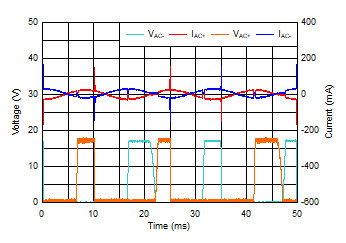Figure 24. The Device VAC Input Current With its Two Components

Equation 1 calculates the shunt current ISHUNT, and Equation 2 calculates the peak current IPEAK.

Equation 1. ISHUNT = VAC (MAX) / XCS = VAC (MAX) × 2 × π × ƒ × CS
Equation 2. IPEAK = VSCIN / RS
Equation 3. VSCIN = 4 × (VLDO_OUT (nom) + 0.6 V)

where

• VAC (MAX) is the maximum VAC supply RMS voltage
• XCS is the impedance of the standard CS capacitor to be used in the application
• VSCIN is the rectified DC voltage on the SCIN pin
• RS is the standard RS resistor to be used in the application

The frequency of the shunt activity is uncorrelated to the AC input frequency. Therefore, the standby power must be measured with a power analyzer. Fortunately, using a power analyzer is relatively simple and the measurement setup shown in Figure 25 and Figure 26 can be used to measure the standby power and the output efficiency.

If the application has an upstream current-limit circuit that limits any high-transient input currents, such as surge or hot-plug currents, the requirement for the surge resistor RS can be relaxed. The input transient current-limit circuit allows the RS resistor to be removed, thus significantly improving the standby power and output efficiency because no power loss is dissipated in RS.Figure 25. Standby Power and Output Efficiency Measurement Setup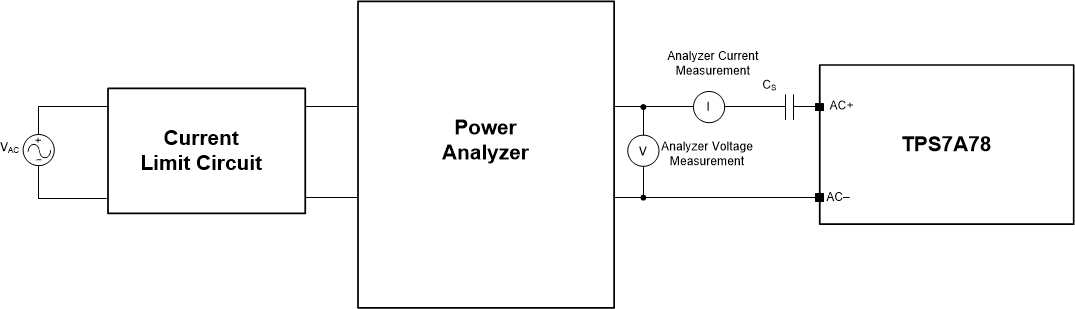Figure 26. Standby Power and Output Efficiency Measurement Setup With an Upstream Current-Limit Circuit

The standby power and output efficiency measurements shown in Figure 27 to Figure 29 were created with the measurement setup in Figure 25.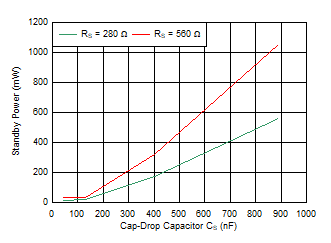VAC = 120 VRMS at 60 Hz, FB, VLDO_OUT = 5.0 V, IOUT = 0 mA
Figure 27. Standby Power vs Cap-Drop Capacitor (CS)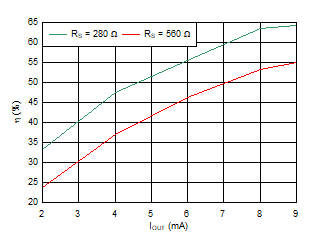VAC = 120 VRMS at 60 Hz, HB, CS = 150 nF, VLDO_OUT = 5.0 V
Figure 29. Efficiency vs IOUT HB Configuration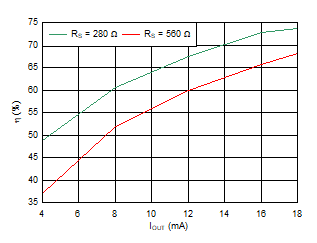VAC = 120 VRMS at 60 Hz, FB, CS = 150 nF, VLDO_OUT = 5.0 V
Figure 28. Efficiency vs IOUT FB Configuration